## Wednesday, 13 February 2013

### Multiplication Squares

In the 2×2 multiplication square below, the boxes at the end of each row and the foot of each column give the result of multiplying the two numbers in that row or column.
 7 5 35 3 4 12 21 20
The 3×3 multiplication square below works in the same way. The boxes at the end of each row and the foot of each column give the result of multiplying the three numbers in that row or column.
 15 108 224 144 8 315
The numbers 19 may be used once and once only.
Can you work out the arrangement of the digits in the square so that the given products are correct?

### Egyptian Rope

The ancient Egyptians were said to make right-angled triangles using a rope which was knotted to make 12 equal sections.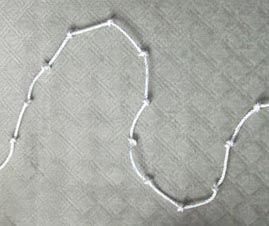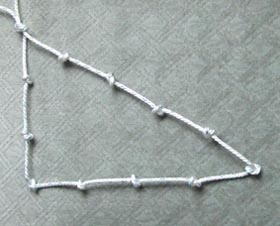If you have a rope knotted like this, what other triangles can you make? (You must have a knot at each corner.)What regular shapes can you make - that is, shapes with equal sides and equal angles?

### Super Shapes

Each of the following shapes has a value: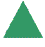=7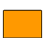=17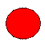=?
The value of the circle changes in each of the following problems.
Can you discover its value in each problem, if the value of the shapes are being added together?
 (a)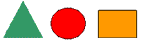=25 (b)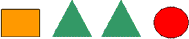=51 (c)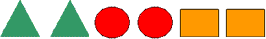=136 (d)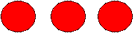=48 (e)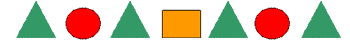=100